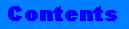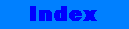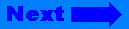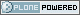# ICTP Portal

##### Sections
« December 2023 »
Su Mo Tu We Th Fr Sa
1 2
3 4 5 6 7 8 9
10 11 12 13 14 15 16
17 18 19 20 21 22 23
24 25 26 27 28 29 30
31

You are here: Home binary_search

# binary_search## binary_search

`Algorithm`

### Summary

Performs a binary search for a value on a container.

None

### Synopsis

```#include <algorithm>

template <class ForwardIterator, class T>
bool
binary_search(ForwardIterator first, ForwardIterator last,
const T& value);

template <class ForwardIterator, class T, class Compare>
bool
binary_search(ForwardIterator first, ForwardIterator last,
const T& value, Compare comp);```

### Description

The binary_search algorithm, like other related algorithms (equal_range, lower_bound and upper_bound) performs a binary search on ordered containers. All binary search algorithms have two versions. The first version uses the less than operator (operator<) to perform the comparison, and assumes that the sequence has been sorted using that operator. The second version allows you to include a function object of type Compare, which it assumes was the function used to sort the sequence. The function object must be a binary predicate.

The binary_search algorithm returns true if a sequence contains an element equivalent to the argument value. The first version of binary_search returns true if the sequence contains at least one element that is equal to the search value. The second version of the binary_search algorithm returns true if the sequence contains at least one element that satisfies the conditions of the comparison function. Formally, binary_search returns true if there is an iterator i in the range [first, last) that satisfies the corresponding conditions:

```!(*i < value) && !(value < *i)
```

or

`comp(*i, value) == false && comp(value, *i) == false`

### Complexity

binary_search performs at most log(last - first) + 2 comparisons.

### Example

```   //
// b_search.cpp
//
#include <vector>
#include <algorithm>
#include <iostream.h>

int main()
{
typedef vector<int>::iterator iterator;
int d1 = {0,1,2,2,3,4,2,2,6,7};
//
// Set up a vector
//
vector<int> v1(d1,d1 + 10);
//
// Try binary_search variants
//
sort(v1.begin(),v1.end());
bool b1 = binary_search(v1.begin(),v1.end(),3);
bool b2 =
binary_search(v1.begin(),v1.end(),11,less<int>());
//
// Output results
//
cout << "In the vector: ";
copy(v1.begin(),v1.end(),
ostream_iterator<int,char>(cout," "));

cout << endl << "The number 3 was "
cout << endl << "The number 11 was "
return 0;
}

Output :
In the vector: 0 1 2 2 2 2 3 4 6 7
The number 3 was FOUND

### Warnings

If your compiler does not support default template parameters, then you need to always supply the Allocator template argument. For instance, you'll have to write:

```vector<int,allocator<int> >
```

`vector<int>`This site conforms to the following standards: